# Entering Expressions in Analyses in Multisim

Publish Date: Mar 27, 2012 | 4 Ratings | 5.00 out of 5 | Print | Submit your review

## Overview

Multisim features a comprehensive suite of SPICE analyses for examining circuit behavior. These analyses range from the basic to sophisticated. Each analysis helps you to obtain valuable information such as the effects of component tolerances and sensitivities. For each analysis you need to set settings that will inform Multisim exactly what to analyze, and how.

Multisim simplifies the procedure for an advanced analysis by providing a configuration window. This abstracts away the complexities associated with SPICE syntax and configuration of an analysis. With this window you merely need to specify the parameter values and output nodes of interest.

This tutorial is part of the National Instruments SPICE Analysis Fundamentals Series. Each tutorial in this series provides you with step-by-step instructions on how to configure and run the different SPICE analyses available in Multisim.

### 1. Introduction

In Multisim you have the ability to add expressions into analyses. The analysis tools in Multisim provide a sophisticated manner in which to study the characteristics of a circuit. For example, in the Transient Analysis window shown in Figure 1, the Output tab displays variables describing node voltages and branch currents in the circuit. These can all be outputted to the Grapher View during the simulation and analysis process.

There are times, however, when you might want to take these variables and combine them into expressions, such as power, to evaluate important characteristics of the circuit. In Multisim this is done easily through the analysis options. In the following example you will perform the simple steps needed to create an expression while performing Transient Analysis on a RF Amplifier circuit.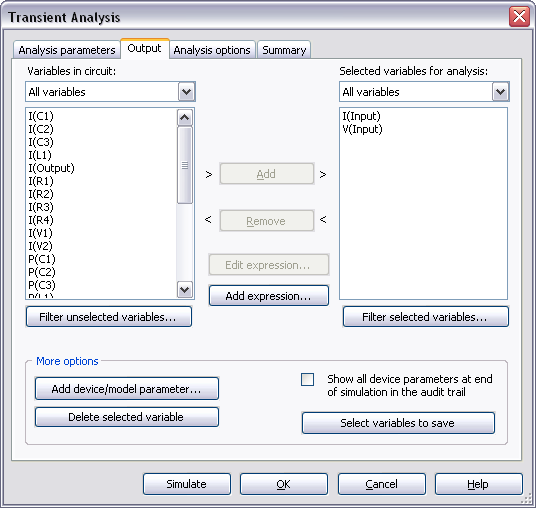Figure 1. Transient Analysis window.

Consider the RF Amplifier circuit shown in Figure 2. The amplifier is configured for maximum output power at 1.5MHz. In this example you will add a custom expression to a Transient Analysis to multiply the input by the output voltages.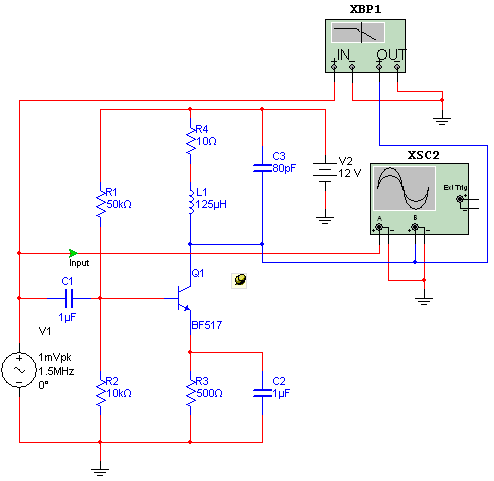Figure 2. RF Amplifier circuit.

1. Select File»Open Samples. The Open file window opens.
2. Open the file RFAmplifier.ms11.
3. Select Simulate»Analyses»Transient. The Transient Analysis window opens.
4. Configure the Analysis Parameters as shown in Figure 3.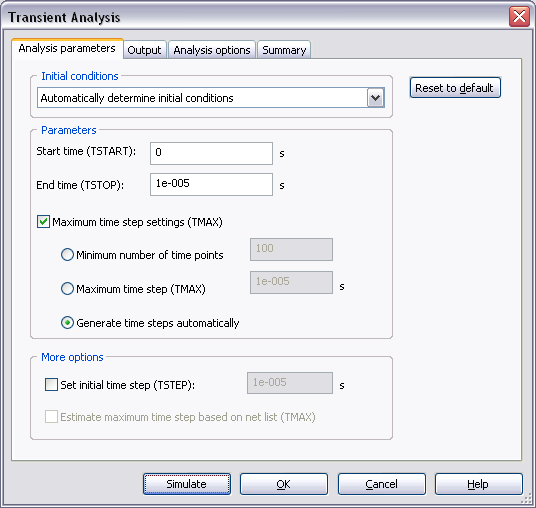Figure 3. Analysis parameters for the Transient Analysis.

Refer to the Transient Analysis tutorial for more details on how to configure the Analysis Parameters tab.

1. Click on the Output tab.

You can now add an expression to analysis. The items in the Variables in circuit list display the voltage and current values which can be grouped into expressions. The output voltage is represented by the variable V(Output) and the voltage taken at the input is represented by the variable V(Input).

1. Click the Add expression button. The Analysis Expression window opens.
2. Select V(Output) from the Variables list and click on Copy variable to expression.
3. Select the * symbol from the Functions list and click on Copy function to expression.
5. Click Copy variable to expression. The Analysis Expression window will look as shown below: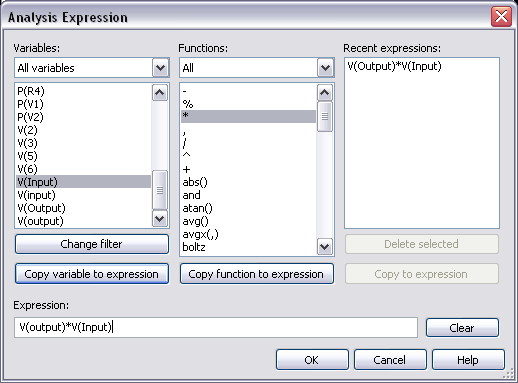Figure 4. Analysis expression window.

1. Click the OK button. You will notice the expression now appears in the Selected variables for analysis list as shown in the next figure: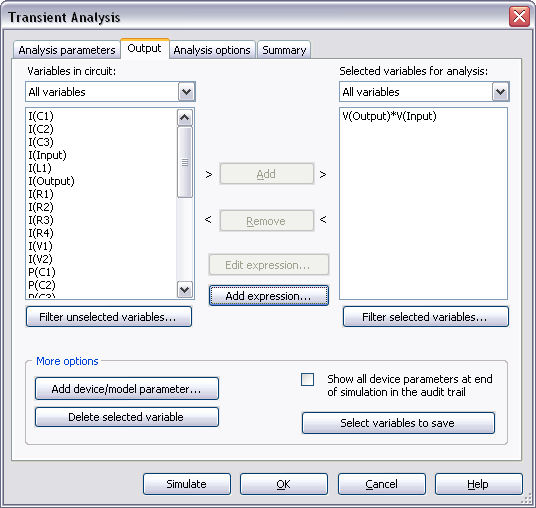Figure 5. Output expression for the Transient Analysis.

1. Click Simulate. The Grapher View window opens. Results are displayed in Figure 6.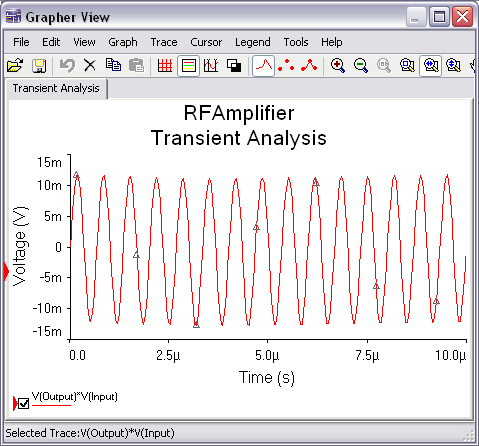Figure 6. Transient Analysis results.

SPICE Analysis Fundamentals

Circuit Design Technical Library